# Introduction

afex_plot() visualizes results from factorial experiments and, more generally, data set with interactions of categorical/factor variables. It does so by combining estimated marginal means and uncertainties associated with these means in the foreground with a depiction of the raw data in the background. If models include continuous covariates, other approaches are recommended (e.g., such as implemented in package effects or by using the possibility of afex_plot to return the data and build the plot on ones own).

This document provides an overview of the different models supported by afex_plot() in addition to the afex objects (i.e., afex_aov and mixed). In general, these are models which are supported by the emmeans package as the afex_plot.default() method uses emmeans to get the estimated marginal means. afex_plot.default() then guesses whether there are repeated measures or all samples are independent. Based on this guess (which can be changed via the id argument) data in the background is plotted. Calculation of error bars can also be based on this guess (but the default is to plot the model based error bars obtained from emmeans).

For a generally introduction to the functionality of afex_plot see: afex_plot: Publication Ready Plots for Experimental Designs

Throughout the document, we will need afex as well as ggplot2. In addition, we load cowplot for function plot_grid() (which allows to easily combine multiple ggplot2 plots). In addition, we will set a somewhat nicer ggplot2 theme.

library("afex")
library("ggplot2")
library("cowplot")
theme_set(theme_bw(base_size = 14) +
theme(legend.position="bottom",
panel.grid.major.x = element_blank(),
panel.grid.minor.x = element_blank()))

Importantly, we also set the contrasts for the current R session to sum-to-zero contrasts. For models that include interactions with categorical variables this generally produces estimates that are easier to interpret.

set_sum_contrasts()
## setting contr.sum globally: options(contrasts=c('contr.sum', 'contr.poly'))

Please note, the best way to export a figure is via ggsave() or a similar function call. For Word and similar document formats, png is a good file type, for LaTeX and similar document formats, pdf is a good file type.

# Base R stats models: lm, glm

afex_plot() generally supports models implemeneted via the stats package. Here I show the main model functions that work with independent samples. These models can be passed to afex_plot without specifying additional arguments.

Most importantly, lm models work directly. For those we use the warpbreaks data.

warp.lm <- lm(breaks ~ wool * tension, data = warpbreaks)

Note that afex_plot produces several messages that are shown here as comments below the corresponding calls. Important is maybe that afex_plot assumes all observations (i.e., rows) are independent. This is of course the case here. In addition, for the first plot we are informed that the presence of an interaction may lead to a misleading impression if only a lower-order effect (here a main effect) is shown. This message is produced by emmeans and passed through.

p1 <- afex_plot(warp.lm, "tension")
## dv column detected: breaks
## No id column passed. Assuming all rows are independent samples.
## NOTE: Results may be misleading due to involvement in interactions
p2 <- afex_plot(warp.lm, "tension", "wool")
## dv column detected: breaks
## No id column passed. Assuming all rows are independent samples.
plot_grid(p1, p2)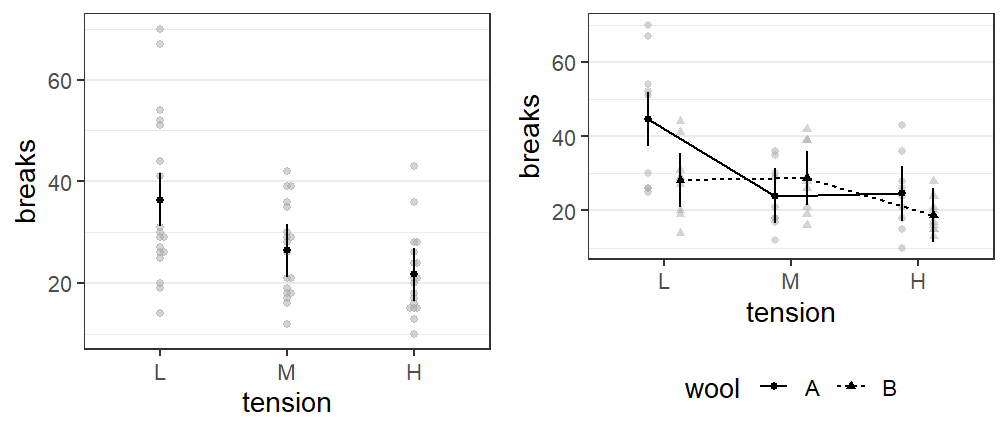glm models also work without further setting. Here we first use a poisson GLM for which we need to generate the data.

ins <- data.frame(
n = c(500, 1200, 100, 400, 500, 300),
size = factor(rep(1:3,2), labels = c("S","M","L")),
age = factor(rep(1:2, each = 3)),
claims = c(42, 37, 1, 101, 73, 14))

We can then fit the data and pass the model object as is.

ins.glm <- glm(claims ~ size + age + offset(log(n)),
data = ins, family = "poisson")
afex_plot(ins.glm, "size", "age")
## dv column detected: claims
## No id column passed. Assuming all rows are independent samples.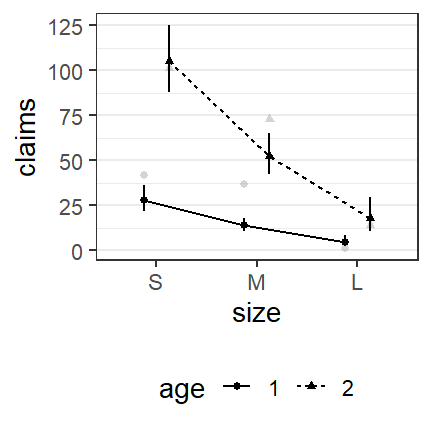afex_plot also works with binomial GLMs for which we also first need to generate some data which we will then fit.

## binomial glm adapted from ?predict.glm
ldose <- factor(rep(0:5, 2))
numdead <- c(1, 4, 9, 13, 18, 20, 0, 2, 6, 10, 12, 16)
sex <- factor(rep(c("M", "F"), c(6, 6)))
SF <- numdead/20  ## dv should be a vector, no matrix
budworm.lg <- glm(SF ~ sex*ldose, family = binomial,
weights = rep(20, length(numdead)))

For this model, we will produce three plots we can then compare. The first only shows the main effect of one variable (ldose). The other show the interaction of the two variables. Because for binomial GLMs we then only have one data point (with several observations), the individual data points and mean cannot be distinguished. This is made clear in the ther two (panels B and C).

a <- afex_plot(budworm.lg, "ldose")
## dv column detected: SF
## No id column passed. Assuming all rows are independent samples.
## NOTE: Results may be misleading due to involvement in interactions
b <- afex_plot(budworm.lg, "ldose", "sex") ## data point is hidden behind mean!
## dv column detected: SF
## No id column passed. Assuming all rows are independent samples.
c <- afex_plot(budworm.lg, "ldose", "sex",
data_arg = list(size = 4, color = "red"))
## dv column detected: SF
## No id column passed. Assuming all rows are independent samples.
plot_grid(a, b, c, labels = "AUTO", nrow = 1)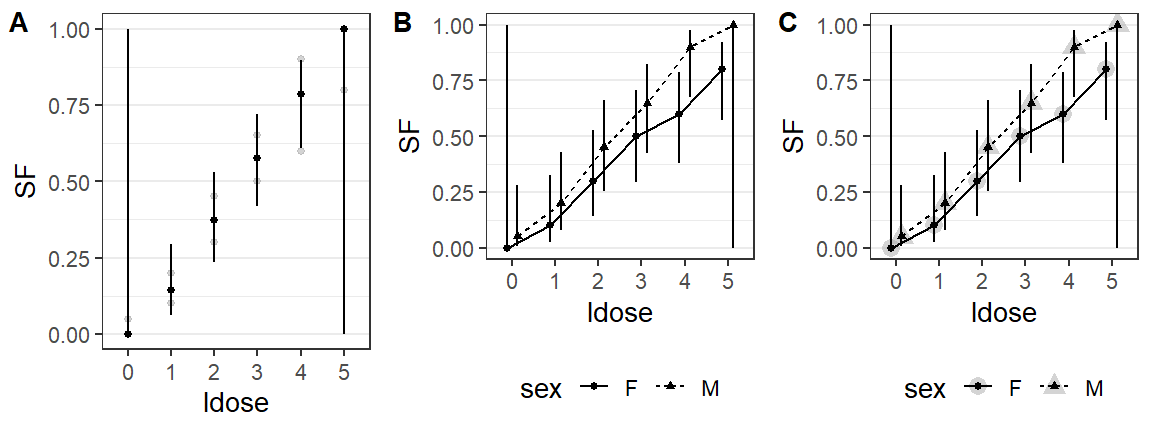# nlme mixed model

Hot to use afex_plot for mixed models fitted with afex::mixed (or lme4 directly) is shown in the other vignette. However, we can also use afex_plot for mixed models fitted with the older nlme package. For this, however we need to pass the data used for fitting via the data argument.

We can change on which of the two nested factors the individual data points in the background are based via the id argument. This is shown below.

## nlme mixed model
data(Oats, package = "nlme")
Oats$nitro <- factor(Oats$nitro)
oats.1 <- nlme::lme(yield ~ nitro * Variety,
random = ~ 1 | Block / Variety,
data = Oats)
plot_grid(
afex_plot(oats.1, "nitro", "Variety", data = Oats), # A
afex_plot(oats.1, "nitro", "Variety", data = Oats), # B
afex_plot(oats.1, "nitro", "Variety", data = Oats, id = "Block"), # C
afex_plot(oats.1, "nitro", data = Oats), # D
afex_plot(oats.1, "nitro", data = Oats, id = c("Block", "Variety")), # E
afex_plot(oats.1, "nitro", data = Oats, id = "Block"), # F
labels = "AUTO"
)
## dv column detected: yield
## No id column passed. Assuming all rows are independent samples.
## dv column detected: yield
## No id column passed. Assuming all rows are independent samples.
## dv column detected: yield
## dv column detected: yield
## No id column passed. Assuming all rows are independent samples.
## NOTE: Results may be misleading due to involvement in interactions
## dv column detected: yield
## NOTE: Results may be misleading due to involvement in interactions
## dv column detected: yield
## NOTE: Results may be misleading due to involvement in interactions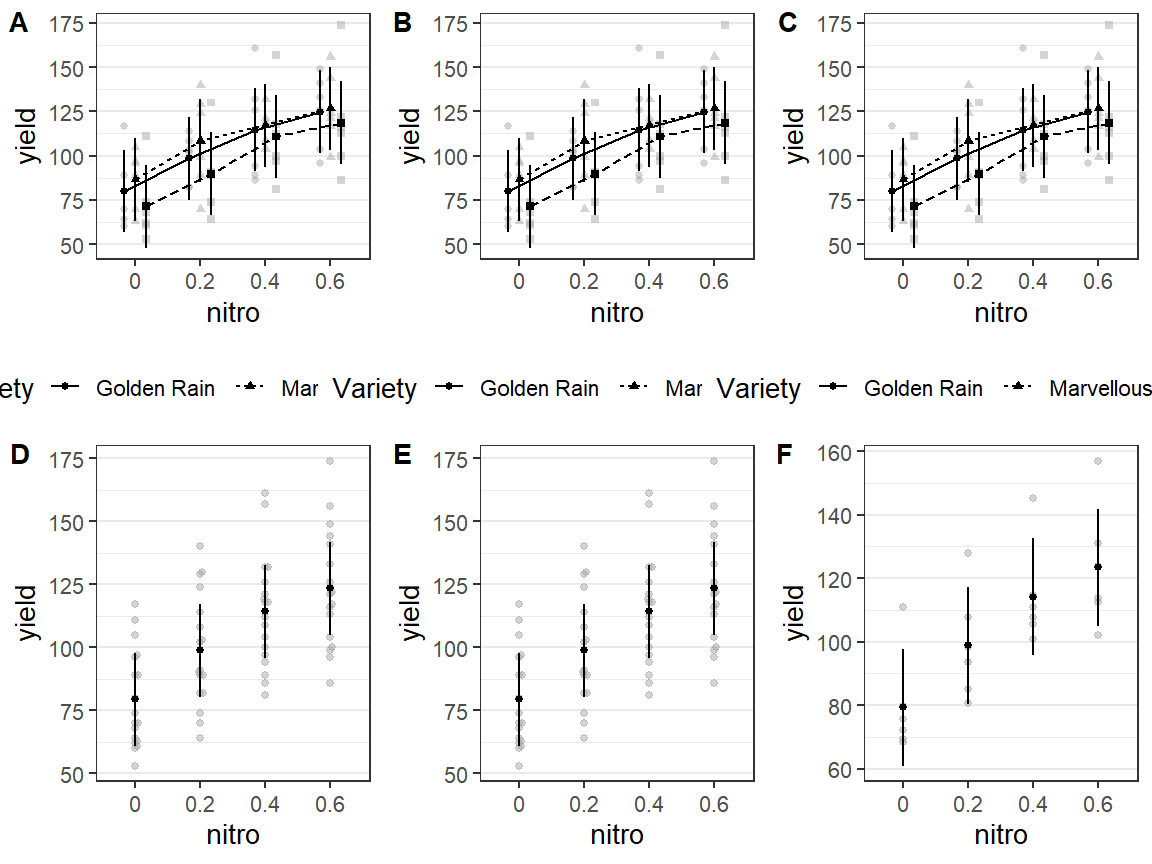# glmmTMB

Support for glmmTMB is also provided. Here we use an example data set for which we model zero-inflation as well as overdispersion. The latter is achieved with a variant of the negative binomial distribution.

library("glmmTMB")
tmb <- glmmTMB(count~spp * mined + (1|site),
ziformula = ~spp * mined,
family=nbinom2, Salamanders)

afex_plot does not automatically detect the random-effect for site. This means that per default all 644 data points are shown. When plotting only one variable, in which the default data_geom is ggbeeswarm::geom_beeswarm, this can lead to rather ugly plots due to the zero inflation. This is shon in panel A below. In panel B, we address this by changing the geom to a violin plot. In panel C, we address this by aggregating the data within site, but still use the beeswarm plot. Note that for panel C it is necessary to pass the data via the data argument as otherwise site cannot be found for aggregation.

plot_grid(
afex_plot(tmb, "spp"),
afex_plot(tmb, "spp", data_geom = geom_violin),
afex_plot(tmb, "spp", id = "site", data = Salamanders),
labels = "AUTO", nrow = 1
)
## dv column detected: count
## No id column passed. Assuming all rows are independent samples.
## NOTE: Results may be misleading due to involvement in interactions
## dv column detected: count
## No id column passed. Assuming all rows are independent samples.
## NOTE: Results may be misleading due to involvement in interactions
## dv column detected: count
## NOTE: Results may be misleading due to involvement in interactions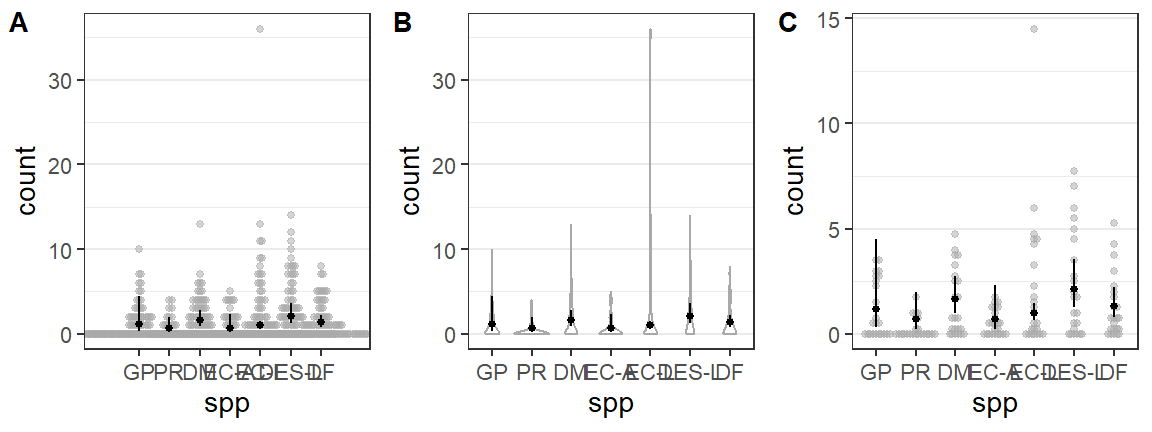When plotting both variables, the problem is somewhat hidden, because instead of beeswarm plots, semi-transparency (i.e., alpha < 1) is used to show overlapping points. In panel B we again make this clearer but this time by adding jitter (on both the y- and x-axis) and increasing the degree of semi-transparancy (i.e., decreasing alpha).

a <- afex_plot(tmb, "spp", "mined")
## dv column detected: count
## No id column passed. Assuming all rows are independent samples.
b <- afex_plot(tmb, "spp", "mined", data_alpha = 0.3,
data_arg = list(
position =
ggplot2::position_jitterdodge(
jitter.width = 0.2,
jitter.height = 0.5,
dodge.width = 0.5  ## needs to be same as dodge
),
color = "darkgrey"))
## dv column detected: count
## No id column passed. Assuming all rows are independent samples.
plot_grid(a, b, labels = "AUTO")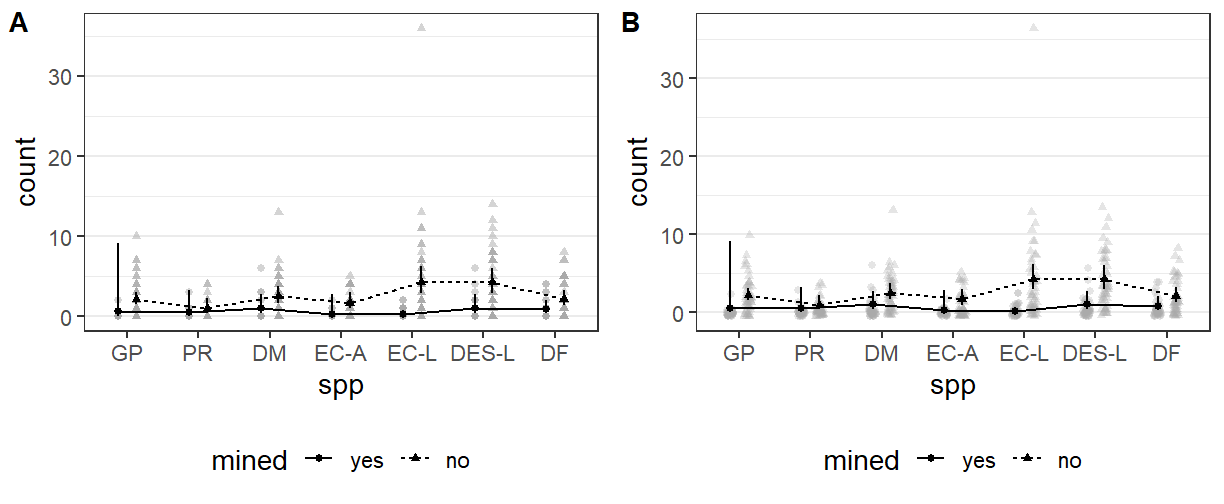For the final plot we also plot the interaction, but this time aggregate the individual-data within site. This allows us again to use a beeswarm plot (after decreasing the width of the “bees”) and produces a relatively clear result.

afex_plot(tmb, "spp", "mined", id = "site", data = Salamanders,
data_geom = ggbeeswarm::geom_beeswarm,
data_arg = list(dodge.width = 0.5, cex = 0.4,
color = "darkgrey")
)
## dv column detected: count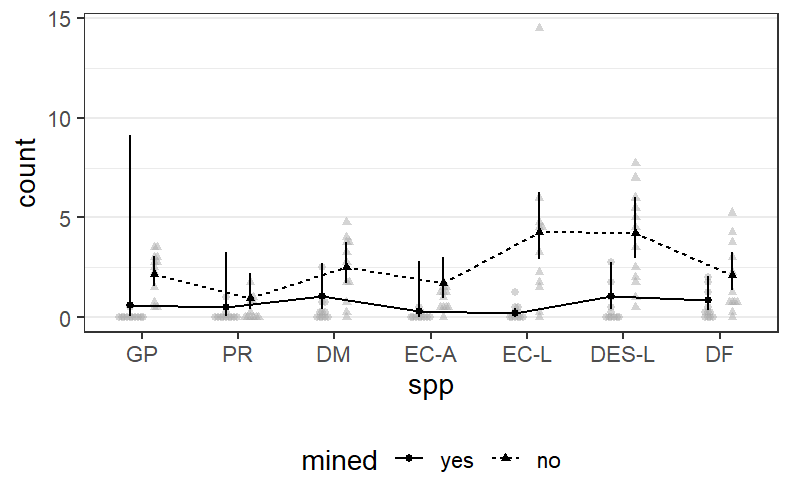# rstanarm

afex_plot() also supports Bayesian models that are also supported via emmeans. For example, we can easily fit a binomial model with rstanarm.

library("rstanarm") ## requires resetting the ggplot2 theme
theme_set(theme_bw(base_size = 14) +
theme(legend.position="bottom",
panel.grid.major.x = element_blank(),
panel.grid.minor.x = element_blank()))
cbpp <- lme4::cbpp
cbpp$prob <- with(cbpp, incidence / size) example_model <- stan_glmer(prob ~ period + (1|herd), data = cbpp, family = binomial, weight = size, chains = 2, cores = 1, seed = 12345, iter = 500) We can directly pass this model to afex_plot. However, we also see quite some zeros leading to a not super nice plot. It looks a bit better using a violin plot for the raw data. b1 <- afex_plot(example_model, "period") ## dv column detected: prob ## No id column passed. Assuming all rows are independent samples. b2 <- afex_plot(example_model, "period", data_geom = geom_violin) ## dv column detected: prob ## No id column passed. Assuming all rows are independent samples. plot_grid(b1, b2, labels = "AUTO")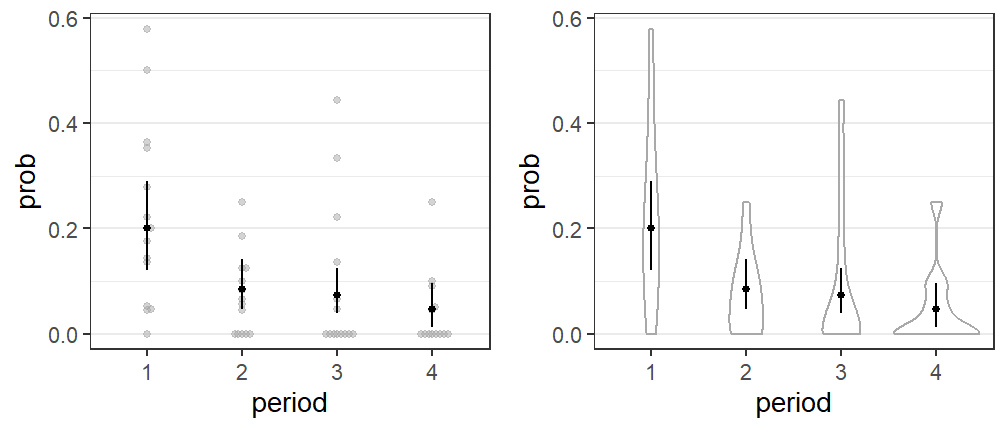We can also produce a plot based on the individual Bernoulli observations in the data. For this, we first need to expand the data such that we have one row per observation. With this, we can then fit the essentially same model as above. cbpp_l <- vector("list", nrow(cbpp)) for (i in seq_along(cbpp_l)) { cbpp_l[[i]] <- data.frame( herd = cbpp$herd[i],
period = cbpp$period[i], incidence = rep(0, cbpp$size[i])
)
cbpp_l[[i]]$incidence[seq_len(cbpp$incidence[i])] <- 1
}
cbpp_l <- do.call("rbind", cbpp_l)
cbpp_l$herd <- factor(cbpp_l$herd, levels = levels(cbpp$herd)) cbpp_l$period <- factor(cbpp_l$period, levels = levels(cbpp$period))
example_model2 <- stan_glmer(incidence ~ period + (1|herd),
data = cbpp_l, family = binomial,
chains = 2, cores = 1, seed = 12345, iter = 500)

Again, this model can be directly passed to afex_plot. However, here we see even more 0 as the data is not yet aggregated. Consequently, we need to pass id = "herd" to aggregate the individual observations within each herd.

b3 <- afex_plot(example_model2, "period")
## dv column detected: incidence
## No id column passed. Assuming all rows are independent samples.
b4 <- afex_plot(example_model2, "period", id = "herd")
## dv column detected: incidence
plot_grid(b3, b4, labels = "AUTO")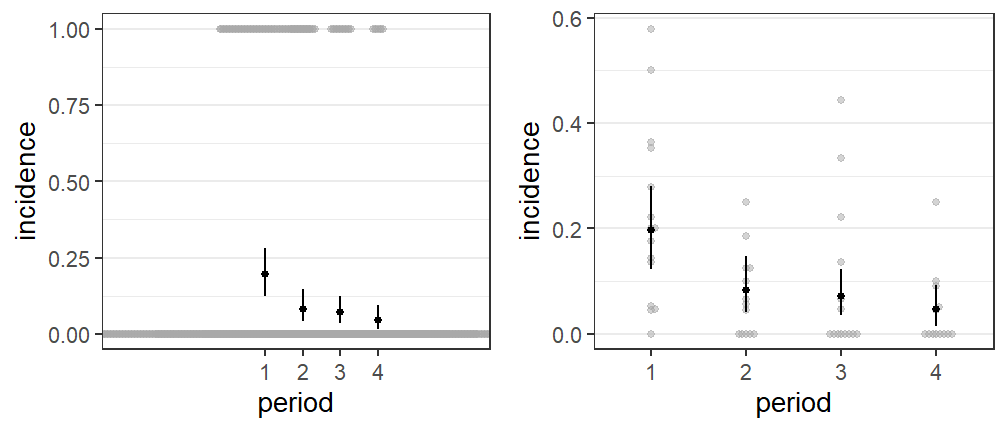We can of course also fit a model assuming a normal distribution using rstanarm. For example using the Machines data.

data("Machines", package = "MEMSS")
mm <- stan_lmer(score ~ Machine + (Machine|Worker), data=Machines,
chains = 2, cores = 1, seed = 12345, iter = 500)

As before, we can pass this model directly to afex_plot (see panel A). However, the data is again not aggregated within the grouping variable Worker. If we want to aggregate the individual data points for the grouping factor, we need to pass both the name of the grouping variable (Worker) and the data used for fitting.

b5 <- afex_plot(mm, "Machine")
## dv column detected: score
## No id column passed. Assuming all rows are independent samples.
b6 <- afex_plot(mm, "Machine", id = "Worker")
## dv column detected: score
plot_grid(b5, b6, labels = "AUTO")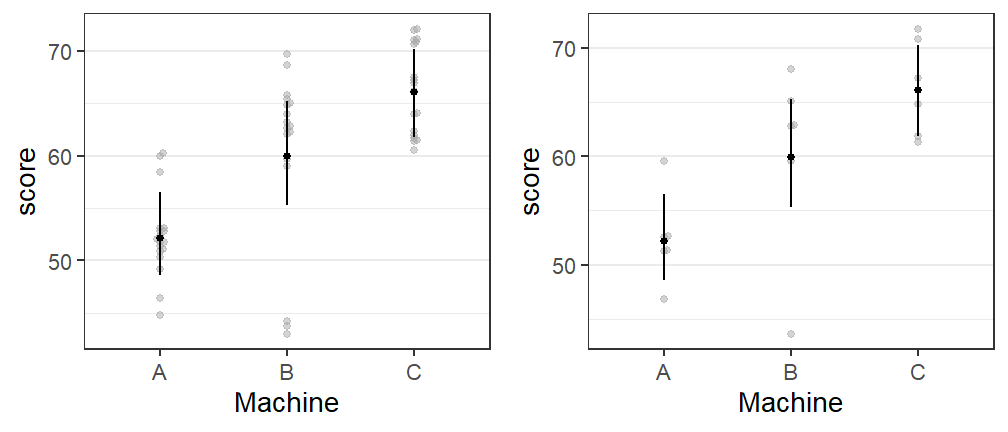# brms

We can also fit the Machines data using brms.

library("brms")
data("Machines", package = "MEMSS")
mm2 <- brm(score ~ Machine + (Machine|Worker), data=Machines,
chains = 2, cores = 1, seed = 12345, iter = 500)

However, to pass a brms object to afex_plot we need to pass both, the data used for fitting as well as the name of the dependent variable (here score) via the dv argument. We again build the plot such that the left panel shows the raw data without aggregation and the right panel shows the data aggregated within the grouping factor Worker.

bb1 <- afex_plot(mm2, "Machine", data = Machines, dv = "score")
## No id column passed. Assuming all rows are independent samples.
bb2 <- afex_plot(mm2, "Machine", id = "Worker",
data = Machines, dv = "score")
plot_grid(bb1, bb2)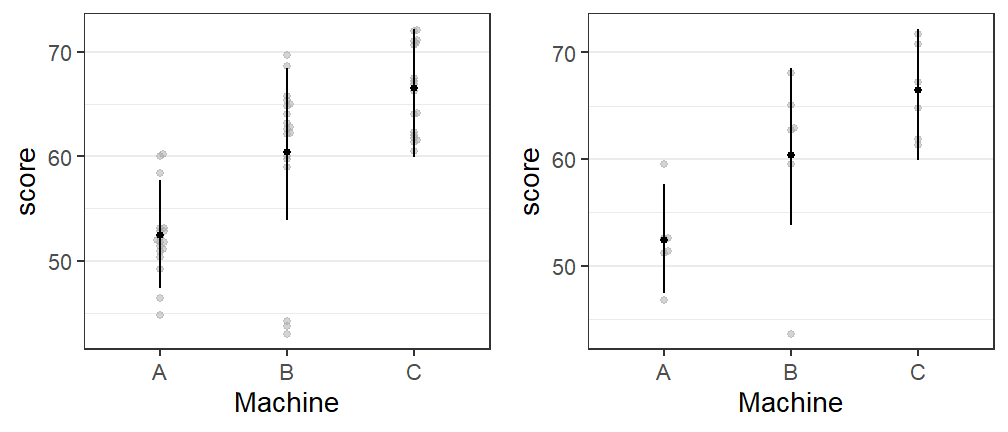Some models are unfortunately not yet supported. For example, models fit with the new and pretty cool looking GLMMadaptive package using some of the special families do not seem to produce reasonable results. The following unfortunately does not produce a reasonable plot.
library("GLMMadaptive")
afex_plot(gm1, "spp", data = Salamanders)